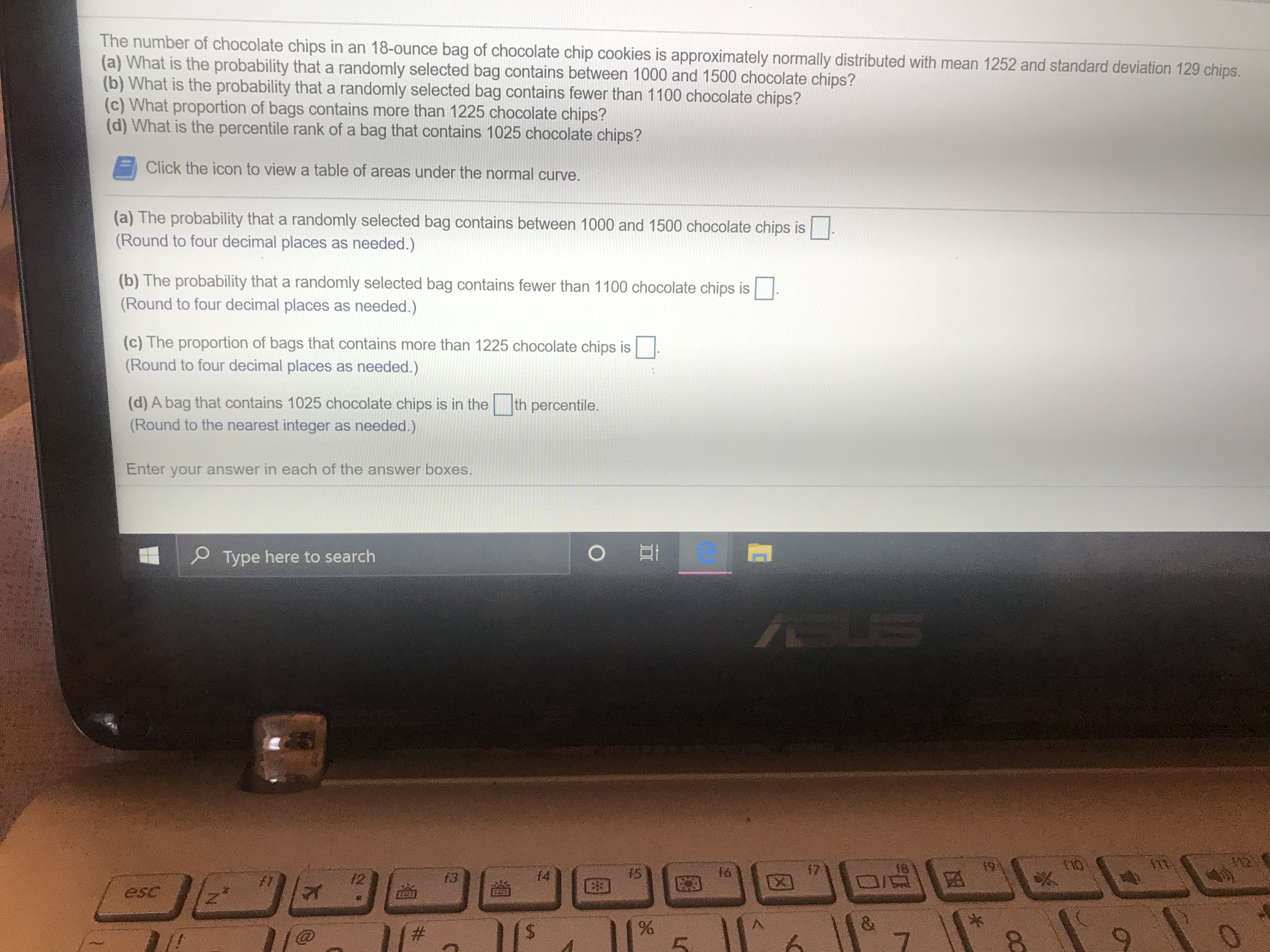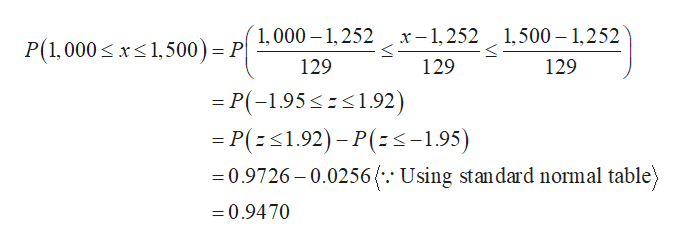# The number of chocolate chips in an 18-ounce bag of chocolate chip cookies is approximately normally distributed with mean 1252 and standard deviation 129 chips.(a) What is the probability that a randomly selected bag contains between 1000 and 1500 chocolate chips?(b) What is the probability that a randomly selected bag contains fewer than 1100 chocolate chips?(c) What proportion of bags contains more than 1225 chocolate chips?(d) What is the percentile rank of a bag that contains 1025 chocolate chips?Click the icon to view a table of areas under the normal curve.(a) The probability that a randomly selected bag contains between 1000 and 1500 chocolate chips is(Round to four decimal places as needed.)(b) The probability that a randomly selected bag contains fewer than 1100 chocolate chips is(Round to four decimal places as needed.)(c) The proportion of bags that contains more than 1225 chocolate chips is(Round to four decimal places as needed.)th percentile.(d) A bag that contains 1025 chocolate chips is in the(Round to the nearest integer as needed.)Enter your answer in each of the answer boxes.Type here to searchUS5tIO\$91715f61413f1esc&7AA\$96

Question
718 viewshelp_outlineImage TranscriptioncloseThe number of chocolate chips in an 18-ounce bag of chocolate chip cookies is approximately normally distributed with mean 1252 and standard deviation 129 chips. (a) What is the probability that a randomly selected bag contains between 1000 and 1500 chocolate chips? (b) What is the probability that a randomly selected bag contains fewer than 1100 chocolate chips? (c) What proportion of bags contains more than 1225 chocolate chips? (d) What is the percentile rank of a bag that contains 1025 chocolate chips? Click the icon to view a table of areas under the normal curve. (a) The probability that a randomly selected bag contains between 1000 and 1500 chocolate chips is (Round to four decimal places as needed.) (b) The probability that a randomly selected bag contains fewer than 1100 chocolate chips is (Round to four decimal places as needed.) (c) The proportion of bags that contains more than 1225 chocolate chips is (Round to four decimal places as needed.) th percentile. (d) A bag that contains 1025 chocolate chips is in the (Round to the nearest integer as needed.) Enter your answer in each of the answer boxes. Type here to search US 5 tIO \$9 17 15 f6 14 13 f1 esc & 7 AA \$ 96 fullscreen
check_circle

Step 1

Hey there! Thank you for posting the question. Since your question has more than 3 parts, we are solving the first 3 parts for you, according to our policy. If you need help with any of the other parts, please re-post the question and mention the part you want answered.

Step 2

It is given that the mean and standard deviation are 1,252 and 129, respectively.

a)

The probability that a randomly selected bag contains between 1,000 and 1,500 chocolate chips is obtained as follows:help_outlineImage Transcriptionclosex-1,252 1,500 - 1,252 1,000 1,252 P(1,000xs 1,500) = P 129 129 129 P(-1.95s1.92) -P(1.92)-P(<-1.95) 0.9726-0.0256(.: Using standard normal table) =0.9470 fullscreen
Step 3

b)

The probability that a randomly selected bag contains fewer...

### Want to see the full answer?

See Solution

#### Want to see this answer and more?

Solutions are written by subject experts who are available 24/7. Questions are typically answered within 1 hour.*

See Solution
*Response times may vary by subject and question.
Tagged in
MathStatistics